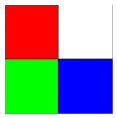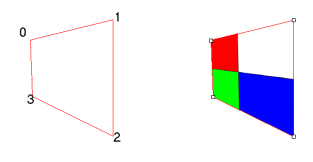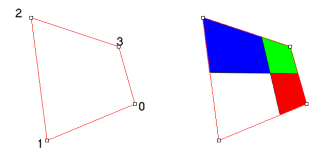←Select platform
In This Topic ▼

# CombineWarpCommand Class

Summary

Combines image data from the source image (the slave) and the destination image (the master), using a perspective warp.

Syntax
C#
VB
Objective-C
C++
``public class CombineWarpCommand : RasterCommand ``
````Public Class CombineWarpCommand  `
`   Inherits RasterCommand ````
``@interface LTCombineWarpCommand : LTRasterCommand ``
``public ref class CombineWarpCommand : public RasterCommand   ``

Remarks

You can warp the entire source image, or a portion of the source image. To warp a portion of the source image, set SourceRectangle accordingly. To warp the entire source image, set the following:

``CombineWarp.Command.SourceRectangle = new LeadRect(0, 0, RasterImage.Width, RasterImage.Height);``

The warp area in the destination image is specified in SetDestinationPoints. The polygon specified in SetDestinationPoints must:

• Be concave
• Fit entirely on the destination bitmap

If either of these conditions is false, this class will throw an exception.

The ordering of the points in SetDestinationPoints can affect the resulting warp. For example, if the source image is the following:then ordering the points in SetDestinationPoints as shown below results in the following warp:while ordering the points in SetDestinationPoints as shown below, results in the following warp:The CombineWarpCommand works by combining the image in the DestinationImage property with the image passed to the Run method. The DestinationImage will then hold the result of the combine operation..

This command does not support signed data images.

Example

This example will warp combines two images.

C#
VB
````using Leadtools; `
`using Leadtools.Codecs; `
`using Leadtools.ImageProcessing; `
`using LeadtoolsExamples.Common; `
` `
`public void CombineWarpCommandExample() `
`{ `
`   RasterCodecs codecs = new RasterCodecs(); `
` `
`   string srcFileName1 = Path.Combine(ImagesPath.Path, "Image1.cmp"); `
`   string srcFileName2 = Path.Combine(ImagesPath.Path, "Image2.cmp"); `
`   string destFileName = Path.Combine(ImagesPath.Path, "CombineWarpCommand.bmp"); `
` `
`   // Load the source images from disk `
`   RasterImage parentImage = codecs.Load(srcFileName1); `
`   RasterImage childImage = codecs.Load(srcFileName2); `
` `
`   // Combine them `
`   CombineWarpCommand command = new CombineWarpCommand(); `
` `
`   LeadPoint[] destPoints = `
`   { `
`      new LeadPoint(100,100), `
`      new LeadPoint(200,75), `
`      new LeadPoint(200,250), `
`      new LeadPoint(100,200) `
`   }; `
` `
`   command.DestinationImage = parentImage; `
`   command.SetDestinationPoints(destPoints); `
`   command.SourceRectangle = new LeadRect(0, 0, childImage.Width, childImage.Height); `
`   command.Flags = CombineWarpCommandFlags.Bilinear; `
`   command.Run(childImage); `
` `
`   // Save it to disk `
`   codecs.Save(parentImage, destFileName, RasterImageFormat.Bmp, 24); `
` `
`   // Clean Up `
`   parentImage.Dispose(); `
`   childImage.Dispose(); `
`   codecs.Dispose(); `
`} ````
````Imports Leadtools `
`Imports Leadtools.Codecs `
`Imports Leadtools.ImageProcessing `
` `
`Public Sub CombineWarpCommandExample() `
`   Dim codecs As RasterCodecs = New RasterCodecs() `
` `
`   Dim srcFileName1 As String = Path.Combine(LEAD_VARS.ImagesDir, "Image1.cmp") `
`   Dim srcFileName2 As String = Path.Combine(LEAD_VARS.ImagesDir, "Image2.cmp") `
`   Dim destFileName As String = Path.Combine(LEAD_VARS.ImagesDir, "CombineWarpCommand.bmp") `
` `
`   ' Load the source images from disk `
`   Dim parentImage As RasterImage = codecs.Load(srcFileName1) `
`   Dim childImage As RasterImage = codecs.Load(srcFileName2) `
` `
`   ' Combine them `
`   Dim command As CombineWarpCommand = New CombineWarpCommand() `
` `
`   Dim destPoints As LeadPoint() = {New LeadPoint(100, 100), New LeadPoint(200, 75), New LeadPoint(200, 250), New LeadPoint(100, 200)} `
` `
`   command.DestinationImage = parentImage `
`   command.SetDestinationPoints(destPoints) `
`   command.SourceRectangle = New LeadRect(0, 0, childImage.Width, childImage.Height) `
`   command.Flags = CombineWarpCommandFlags.Bilinear `
`   command.Run(childImage) `
` `
`   ' Save it to disk `
`   codecs.Save(parentImage, destFileName, RasterImageFormat.Bmp, 24) `
` `
`   ' Clean Up `
`   parentImage.Dispose() `
`   childImage.Dispose() `
`End Sub `
` `
`Public NotInheritable Class LEAD_VARS `
`   Public Const ImagesDir As String = "C:\Users\Public\Documents\LEADTOOLS Images" `
`End Class ````
````c#[Silverlight C# Example] `
`using Leadtools; `
`using Leadtools.Codecs; `
`using Leadtools.Examples; `
`using Leadtools.ImageProcessing; `
`using Leadtools.Windows.Media; `
` `
`//public void CombineWarpCommandExample(RasterImage parentImage, RasterImage childImage, Stream destStream) `
`//{ `
`//   RasterCodecs codecs = new RasterCodecs(); `
` `
`//   // Combine them `
`//   CombineWarpCommand command = new CombineWarpCommand(); `
` `
`//   Point[] destPoints = `
`//   { `
`//      new Point(100,100), `
`//      new Point(200,75), `
`//      new Point(200,250), `
`//      new Point(100,200) `
`//   }; `
` `
`//   command.DestinationImage = parentImage; `
`//   command.SetDestinationPoints(destPoints); `
`//   command.SourceRectangle = new LeadRect(0, 0, childImage.Width, childImage.Height); `
`//   command.Flags = CombineWarpCommandFlags.Bilinear; `
`//   command.Run(childImage); `
` `
`//   // Save it to disk `
`//   codecs.Save(parentImage, destStream, RasterImageFormat.Bmp, 24); `
` `
`//   // Clean Up `
`//   parentImage.Dispose(); `
`//   childImage.Dispose(); `
`//} `
`vb[Silverlight VB Example] `
`Imports Leadtools `
`Imports Leadtools.Codecs `
`Imports Leadtools.ImageProcessing `
`Imports Leadtools.Windows.Media `
` `
`'public void CombineWarpCommandExample(RasterImage parentImage, RasterImage childImage, Stream destStream) `
`'{ `
`'   RasterCodecs codecs = new RasterCodecs(); `
` `
`'   // Combine them `
`'   CombineWarpCommand command = new CombineWarpCommand(); `
` `
`'   Point[] destPoints = `
`'   { `
`'      new Point(100,100), `
`'      new Point(200,75), `
`'      new Point(200,250), `
`'      new Point(100,200) `
`'   }; `
` `
`'   command.DestinationImage = parentImage; `
`'   command.SetDestinationPoints(destPoints); `
`'   command.SourceRectangle = new LeadRect(0, 0, childImage.Width, childImage.Height); `
`'   command.Flags = CombineWarpCommandFlags.Bilinear; `
`'   command.Run(childImage); `
` `
`'   // Save it to disk `
`'   codecs.Save(parentImage, destStream, RasterImageFormat.Bmp, 24); `
` `
`'   // Clean Up `
`'   parentImage.Dispose(); `
`'   childImage.Dispose(); `
`'} ````

Requirements